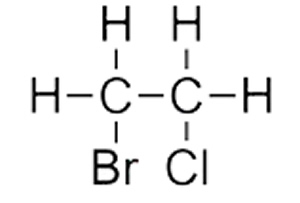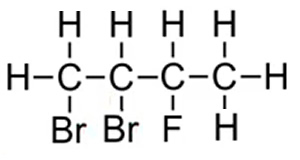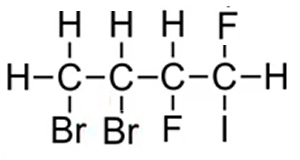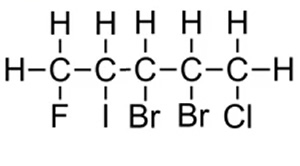Naming haloalkanes Hydrocarbons in which one or more hydrogens are replaced by one or more halogens are known as haloalkanes. Cl = Chloro Br =Bromo F = Fluoro I = Iodo Naming them is s straight forward procedure if we follwo the steps below. Step 1 We identify the longest carbon chain in the molecule. Step 2 Number the carbons so that the halogens are on the lowest carbon number possible. Step 3 If the molecule contains a halogen on both ends then carbon number one is given to the carbon with the halogen that comes first aphabetically. Examples 1 Consider the molecule on the right. Step 1 We identify that the longest carbon chain is ethane. Step 2 We see that a halogen is on each carbon. We therefore give the carbon that bromine is attached the lowest number since bromo comes before chloro alphabetically. The compound is thus named 1-bromo-2-chloroethaneExamples 1 Consider the molecules on the right. Step 1 We identify that the longest carbon chain is butane. Step 2 We number each carbon so that the halogens are on the lowest numbered carbons. We therefore give the carbon that bromine is attached the lowest number . The compound is thus named 1,2-dibromo-3-fluorobutaneThe compound is thus named 3,4-dibromo-1,2-difuoro-1-iodobutane as this gives the lowest sum of carbons 3 + 4 + 1+ 2 + 1 = 11. If we had numbered the carbons from the right the name would have been 1,2-dibromo-3,4-difuoro-4-iodobutane and this would have given the highest sum of carbons 1 + 2 +3 + 4+ 4 = 14.Now see if you can name the compound on the right. Whether we number the carbons from left to right or right to left it doesnt matter we still get the same total (15) . Hence we go by alphabetical order of the halogens. Chloro comes before fluoro. 1-chloro-2,3-dibromo-4-iodo-5-fluoropentaneHome